Wonderlic : Computation

Example Questions

Example Question #3 : Mathematical Functions And Reasoning

Five chocolate baklavas cost sixty-eight dollars. How much would fifteen chocolate baklavas cost?

136 dollars

112 dollars

180 dollars

204 dollars

204 dollars

Explanation:

To answer this question you could have simply multiplied 68 by 3 and gotten 204. Or, to do this question even quicker, you could have looked at the last digits. Knowing that 3 multiplied by 8 is 4, you would know that the final digit of the answer would be four, and the only answer that ended with a 4 was the correct answer, 204 dollars.

Example Question #4 : Mathematical Functions And Reasoning

The marketing team budgeted $1,756 for raises this year. Thus far they have given out$428. How much of the money budgeted for raises remains?

$1328$1456

$1330$1398

$1328 Explanation: This question calls for simple arithmetic. One must simply subtract 1756 from 428. One can see this answer quickly by recognizing that the correct answer will end with the digits 28 (56 minus 28 is 28). Example Question #5 : Mathematical Functions And Reasoning A small pile of loose pencil shavings was assessed as having a value of$4500, the three close friends (Julio, DeMare, and Aiko) who found the pile of pencil shavings decided to divide the money based on how much of the shavings blew, as chance and the breath of the wind had dictated, onto the legs of their pants. 10% of the shavings wound up on Aiko's pants, 15% on Julio's, and 75% on DeMare's. How much more money did DeMare take than Aiko?

$3375$3000

$2925$450

$2925 Explanation: 75% of 4500 is 3375, so DeMare made$3375 from the pencil shavings. Aiko only made $450. All you have to do now is subtract 450 from 3375. Example Question #6 : Mathematical Functions And Reasoning Which is equal to the radius of a circle with area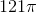? Possible Answers:Correct answer:Explanation: The formula for the areaof a circle, given its radius, is. Replacewith: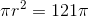To find the radius, first, divide both sides by: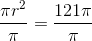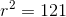Now, find the square root of both sides. Since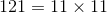, 11 is the square root of 121, so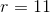. The radius of the given circle is 11. Example Question #1 : Computation Andy, Sandy, and Mandy go in on a pizza. The total cost of the pizza is$14. Andy contributes half the cost, and Sandy contributes $3. What will Mandy contribute? Possible Answers: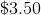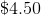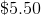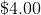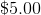Correct answer:Explanation: Andy contributes half the cost of the pizza. The pizza costs$14.00, so his share of the cost is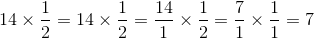;

that is, $7.00. Sandy contributes$3.00, leaving Mandy to contribute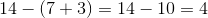;

that is, \$4.00.

Example Question #2 : Computation

Square 0.7.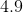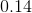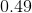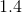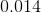Explanation:

To square a number means to multiply it by itself, so we seek to compute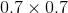To multiply two decimal fractions, first multiply, ignoring the decimal points:Move the (implied) decimal point the number of spaces right equal to the total number of digits right of the decimal points in the factors; this can be seen to be two: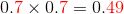The product is 0.49.

Example Question #9 : Mathematical Functions And Reasoning

The first 40 miles of a 100-mile trip took one hour; the whole trip took two hours and fifteen minutes. To the nearest whole, what was the average speed during the remaining 60 miles of the trip?

36 miles per hour

54 miles per hour

60 miles per hour

48 miles per hour

75 miles per hour

48 miles per hour

Explanation:

The average speed during a trip ofmiles over a period ofhours is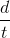miles per hour. Here, the distance driven is 60 miles; the time it took was two hours and fifteen minutes total minus the hour for the first 40 miles, which is one hour and fifteen minutes. Since fifteen minutes is equal to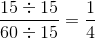hours, we get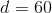and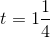Therefore, the average speed traveled during the second part of the trip is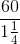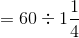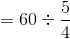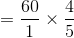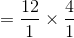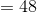miles per hour.

Example Question #10 : Mathematical Functions And Reasoning

Evaluate: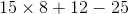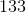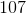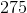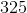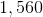Explanation:

In the order of operations, in the absence of grouping symbols, multiplication takes precedence over addition and subtraction. Multiply 15 by 8 first: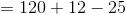Addition and subtraction have equal precedence and are worked in left-to-right order. Add 120 and 12 next: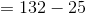Now subtract: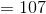,

the correct response.

Example Question #1 : Computation

A square has perimeter 20 feet. Give its area.

400 square feet

20 square feet

80 square feet

5 square feet

25 square feet

25 square feet

Explanation:

The perimeter of a figure is the sum of the lengths of its sides. A square comprises four sides of equal length, so, if the perimeter of the square is 20 feet, then each side has length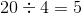feet.

The area of the square is equal to the length of a side multiplied by itself, so the area of this square is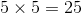square feet.